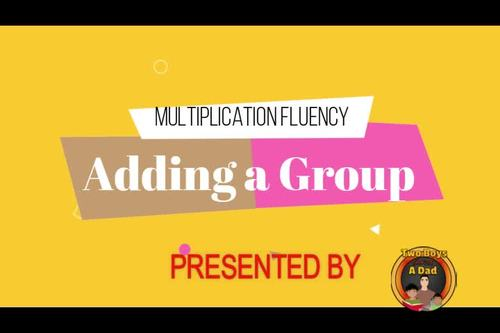# Multiplication Fluency Strategies with Posters, Games and More!Subject
Resource Type
File Type

PDF

(10 MB|126 pages)
Product Rating
4.0
(8 Ratings)
Standards
• Product Description
• StandardsNEW

Learning multiplication facts takes time, but fluency requires using strategies. What happens when students don't recall or have trouble memorizing? They need to learn multiplication fluency strategies. Save time with these ready to use posters, templates, games, worksheets and more.

WHY TEACH FLUENCY STRATEGIES?

Fluency is more than just quick recall. Fluency is the ability to be accurate, efficient and flexible. We can't always memorize everything and we can't always recall with automaticity all the facts. Instead, we need to rely on strategies such as halving and doubling or using known facts. This resource provides strategies, activities, games, practice pages and more to teach and achieve multiplication fluency.

WHAT ARE THE FLUENCY STRATEGIES?

There are two types of strategies: foundational strategies and derivative strategies.

• Foundational strategies including counting by 2s, 5s, 10s, learning the squares (2, 4, 9, 16, etc.), as well as knowing the Identity and Zero Properties of Multiplication.

• Derivative strategies build on foundational strategies. They include halving and doubling, using a nearby square, adding or subtracting a group as well as, the Commutative and Distributive Properties of Multiplication.

WHAT’S INCLUDED

• 16 colorful charts to teach the Foundation Strategies
• 22 colorful charts to teach the Derivative Strategies
• A Sample Lesson Plan for a Foundation Strategy
• A Sample Lesson Plan for a Derivative Strategy
• Sequence for teaching the multiplication tables aligned with strategies
• Support Printables for the Foundation Strategies that include games, flashcards, bookmarks and more
• Support Printables for the Derivative Strategies that include games and templates

HOW IT WORKS

Teach the strategies until the student can use the strategies mentally. Similar to using addition and subtraction mental math strategies such as decomposing a number, compensating, doubles, or doubles plus one, these mental math multiplication strategies can lead the student to multiplication fluency.

Incorporate the resources into any existing math curriculum or program.

• Then use the resources to enhance a multiplication unit of study.
• Start out by teaching explicitly one of the strategies using one of the teaching charts.
• Then use the included templates (derivative strategies only) to have students practice step by step the strategy.
• Follow up with the included games and practice pages.
• Use in whole group, small group or for center practice.

A Sample Lesson Plan gives the teacher an outline on how to focus on one specific strategy. These strategies are taught at any pace the teacher sets which should be dependent on the internalization of the strategies by the student.

Check out a short YouTube Videos of one of the multiplication fluency strategies being used.

As always feedback, suggestions, and comments always welcomed!

********************************************************

Interested in more resources for math for:

★ distributive property

★ multiplication properties

★ multiplication resources

★ math centers

★ math games

★ area and perimeter

★ geometry

********************************************************

♦ Be sure to click on the green ★ to follow me for updates and new product announcements!

♦ Do you need some fresh ideas and tips? Then be sure to visit my My BLOG

♦ Each time you give feedback, TPT gives you feedback credits that you use to lower the cost of your future purchases. I value your feedback greatly!

♦ If you have any questions or concerns, go ahead and contact me through the Q & A and I’ll get back to you ASAP!

********************************************************

Fluently multiply and divide within 100, using strategies such as the relationship between multiplication and division (e.g., knowing that 8 × 5 = 40, one knows 40 ÷ 5 = 8) or properties of operations. By the end of Grade 3, know from memory all products of two one-digit numbers.
Apply properties of operations as strategies to multiply and divide. Examples: If 6 × 4 = 24 is known, then 4 × 6 = 24 is also known. (Commutative property of multiplication.) 3 × 5 × 2 can be found by 3 × 5 = 15, then 15 × 2 = 30, or by 5 × 2 = 10, then 3 × 10 = 30. (Associative property of multiplication.) Knowing that 8 × 5 = 40 and 8 × 2 = 16, one can find 8 × 7 as 8 × (5 + 2) = (8 × 5) + (8 × 2) = 40 + 16 = 56. (Distributive property.)
Total Pages
126 pages
Included
Teaching Duration
N/A
Report this Resource to TpT
Reported resources will be reviewed by our team. Report this resource to let us know if this resource violates TpT’s content guidelines.